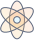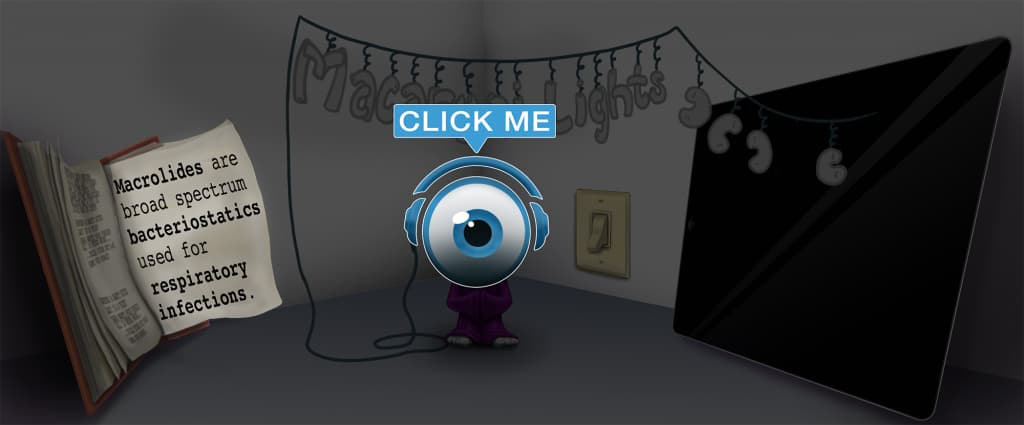# Master Spontaneous vs. Nonspontaneous with Picmonic for College

## With Picmonic, facts become pictures. We've taken what the science shows - image mnemonics work - but we've boosted the effectiveness by building and associating memorable characters, interesting audio stories, and built-in quizzing.

### Spontaneous vs. Nonspontaneous

Spartan vs Nun-SpartanPicmonic
Gibbs free energy measures whether a reaction is spontaneous or not with the equation ΔG = ΔH - TΔS. Thus, based on whether ΔH or ΔS are positive or negative, the reaction may be spontaneous or nonspontaneous at low or high temperatures. It is useful to remember at what temperatures the reaction is spontaneous as written with varying enthalpy and entropy values. If ΔH and ΔS are both negative, the reaction is only spontaneous at low temperatures. This is because at low temperatures the entropy term will be a smaller positive number, making it more likely that the negative enthalpy can drive the spontaneity of the reaction. The opposite is true if ΔH and ΔS are both positive. In this case, a high temperature is needed to make the entropy term a large negative number, because the enthalpy change is positive. If ΔH is negative and ΔS is positive, the reaction is spontaneous at all temperatures because the change in Gibbs free energy is always negative. Contrastingly, if ΔH is positive and ΔS is negative, the reaction is nonspontaneous at all temperatures as written. This is because the change in Gibbs free energy is always positive.
12 KEY FACTS
Spontaneous at Low Temperatures
Spartan in the the Cold

Based on the equation for Gibbs Free Energy, there are certain reactions that are spontaneous at only low temperatures.

ΔH is Negative
Delta-H-Horse Devil

If ΔS, entropy, is negative, and ΔH, enthalpy, is negative, the reaction is spontaneous at low temperatures. Based on the equation ΔG = ΔH - TΔS, we want Gibbs free energy to be negative. This requires a low temperature because a negative change in entropy makes that term positive. If the temperature is high, Gibbs free energy is more likely to be positive.

ΔS is Negative
Delta-S-Snake Devil

If ΔS, entropy, is negative, and ΔH, enthalpy, is negative, the reaction is spontaneous at low temperatures. Based on the equation ΔG = ΔH - TΔS, we want Gibbs free energy to be negative. This requires a low temperature because a negative change in entropy makes that term positive. If the temperature is high, Gibbs free energy is more likely to be positive and the reaction is likely to be nonspontaneous.

Spontaneous at High Temperatures
Spartan in the Heat

Based on the equation for Gibbs Free Energy, there are certain reactions that are spontaneous at only high temperatures.

ΔH is Positive
Delta-H-Horse Angel

If ΔS, entropy, is positive, and ΔH, enthalpy, is positive, the reaction is spontaneous at high temperatures. Based on the equation ΔG = ΔH - TΔS, we want Gibbs free energy to be negative. This requires a high temperature because a positive change in enthalpy makes that term in the equation positive. If the temperature is high. In order for Gibbs free energy to be negative, the entropy term must be greater in magnitude than the enthalpy term, requiring a high temperature.

ΔS is Positive
Delta-S-Snake Angel

If ΔS, entropy, is positive, and ΔH, enthalpy, is positive, the reaction is spontaneous at high temperatures. Based on the equation ΔG = ΔH - TΔS, we want Gibbs free energy to be negative. This requires a high temperature because a positive change in enthalpy makes that term in the equation positive. If the temperature is high. In order for Gibbs free energy to be negative, the entropy term must be greater in magnitude than the enthalpy term, requiring a high temperature.

Spontaneous at All Temperatures
Spartan at All Temperature

Some reactions are spontaneous at all temperatures depending on the values of enthalpy and entropy.

ΔH is Negative
Delta-H-Horse Devil

If ΔS, entropy, is positive, and ΔH, enthalpy, is negative, the reaction is spontaneous at all temperatures. This is because based on the equation ΔG = ΔH - TΔS, Gibbs free energy would always be negative. Thus, the reaction would always be spontaneous.

ΔS is Positive
Delta-S-Snake Angel

If ΔS, entropy, is positive, and ΔH, enthalpy, is negative, the reaction is spontaneous at all temperatures. This is because based on the equation ΔG = ΔH - TΔS, Gibbs free energy would always be negative. Thus, the reaction would always be spontaneous.

Nonspontaneous at All Temperatures
Nun-Spartan at All Temperatures

Some reactions will never be spontaneous as written based on the changes in entropy and enthalpy associated with them.

ΔH is Positive
Delta-H-Horse Angel

If ΔS, entropy, is negative, and ΔH, enthalpy, is positive, the reaction isnon spontaneous at all temperatures. This is because based on the equation ΔG = ΔH - TΔS, Gibbs free energy would always be positive and can never be negative. Thus, the reaction would always be nonspontaneous.

ΔS is Negative
Delta-S-Snake Devil

If ΔS, entropy, is negative, and ΔH, enthalpy, is positive, the reaction isnon spontaneous at all temperatures. This is because based on the equation ΔG = ΔH - TΔS, Gibbs free energy would always be positive and can never be negative. Thus, the reaction would always be nonspontaneous.

Unlock all 12 facts & definitions with Picmonic Free!

## Take the Spontaneous vs. Nonspontaneous Quiz

Picmonic's rapid review multiple-choice quiz allows you to assess your knowledge.

## Picmonic for College CoversCollege
300+ Videos
2,000+ Facts

## Our Story Mnemonics Increase Mastery and Retention

Memorize facts with phonetic mnemonics

Unforgettable characters with concise but impactful videos (2-4 min each)

### Ace Your College Classes & Exams with Picmonic:

#### Over 1,240,000 students use Picmonic’s picture mnemonics to improve knowledge, retention, and exam performance.